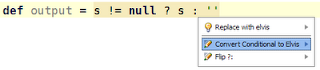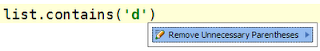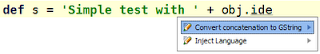## October 22, 2009

### Groovy Intentions in IntelliJ IDEA Community Edition

IntelliJ IDEA Community Edition has a nice feature called intentions. Intentions offer a way to simplify our code. In our editor we can select an intention with Alt+Enter and IntelliJ IDEA shows a popup with available intensions. Let's take a look at some useful intentions when developing Groovy code.```def s = "Simple test \${obj.ide}"

// Becomes:
def s = "Simple test \$obj.ide"
``````def output = s != null ? s : ''

// Becomes:
def output = s ?: ''
``````def list = ['a', 'b', 'c']
for (c in list) {
println c.toUpperCase()
}

// Becomes:
def list = ['a', 'b', 'c']
list.each { c ->
println c.toUpperCase()
}
``````def c = list.getAt(0)

// Becomes:
def c = list
``````def printObj(date, text, list) {
println """
Print on \$date \$text and list has size \${list.size()}
"""
}

// Becomes:
def printObj(params) {
println """
Print on \$params.date \$params.text and list has size \${params.list.size()}
"""
}
``````list.contains('d')

// Becomes:
list.contains 'b'
``````class User { String name }
def u = new User()
u.setName('mrhaki')

// Becomes:
class User { String name }
def u = new User()
u.name = 'mrhaki'
``````def s = 'Simple test with ' + obj.ide

// Becomes:
def s = "Simple test with \$obj.ide"
```